Home

ARTICLE 44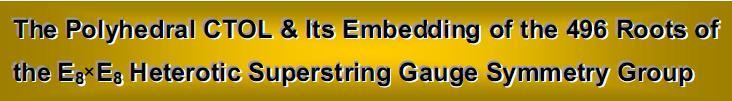by

Stephen M. Phillips

Flat 4, Oakwood House, 117-119 West Hill Road. Bournemouth. Dorset BH2 5PH. England.

Website: http://www.smphillips.mysite.com

Abstract

 Previous articles have presented considerable evidence that correlates the properties of the disdyakis triacontahedron with the inner form of the Tree of Life. It established beyond reasonable doubt that this Catalan solid is the polyhedral counterpart of the latter. Its prescription by the Kabbalistic Divine Names supports this conclusion. Just as the root structure of the heterotic superstring symmetry group E8×E8 is embodied in the inner Tree of Life, so it manifests in the pattern of geometrical elements of the disdyakis triacontahedron. Both its seven layers of vertices and its 15 layers of vertices form sets of polygons with the remarkable attribute of being geometrically analogous to this root structure. As confirmation of their holistic character, the archetypal pattern of Plato’s Lambda Tetractys, which generates the tone ratios of the Pythagorean musical scale, appears in the geometrical composition of the polyhedron and its 15 polygons. The disdyakis triacontahedron is shown to be also the polyhedral representation of the Cosmic Tree of Life (CTOL), which maps the seven cosmic planes of consciousness. Half of the polyhedron maps the cosmic physical plane and its other half maps the six cosmic superphysical planes. The polyhedron provides a simple explanation of why the Hebrew word ‘Malkuth,’ which signifies the physical aspect of the Tree of Life, has the gematria number value 496 and why, as the dimension of E8×E8, it appears at the heart of superstring physics, for this number has a more profound meaning than that understood by researchers in superstring theory. When constructed from tetractyses, the 15 polygons and the polyhedron have 1680 yods surrounding its centre other than its two opposite vertices. This is the number of turns in each helical whorl of the basic unit of matter paranormally described over a century ago by the Theosophists Annie Besant and C. W. Leadbeater. It is also the number of geometrical elements surrounding the axis of the polyhedron that are required to construct it. The number 168 is the gematria number value of the Hebrew name of the Mundane Chakra of Malkuth. The division of the form-building elements into 780 edges and 900 vertices & triangles reproduces the gematria number values 78 and 90 of the Hebrew words making up this name, making unlikely the possibility of coincidence. The vertices, edges and triangles in the polyhedron and its 15 polygons that are not shared with each other reproduce the gematria number value of Tiphareth, the Sephirah at the centre of the Tree of Life. The number of vertices making up both is 137, which is the number that determines the fine-structure constant measuring the strength of the coupling of the electromagnetic field to the electron. This confirms its status as the polyhedral Tree of Life because a number of previous articles have shown that 137 is — like 168 — one of the parameters that define holistic systems.

1

1. Introduction
In Article 27, it was shown that the Catalan solid (1) with the most faces — the disdyakis triacontahedron, which possesses 62 (2) vertices, 180 edges & 120 faces — embodies the superstring structural parameter 1680 discussed in many previous articles. This is because it is the polyhedral version of the universal Tree of Life blueprint governing holistic systems, such as the heterotic superstring described by the Theosophists Annie Besant and C. W. Leadbeater (Fig. 1). When its 180 internal triangles, having the centre of the polyhedron as a vertex and its edges as their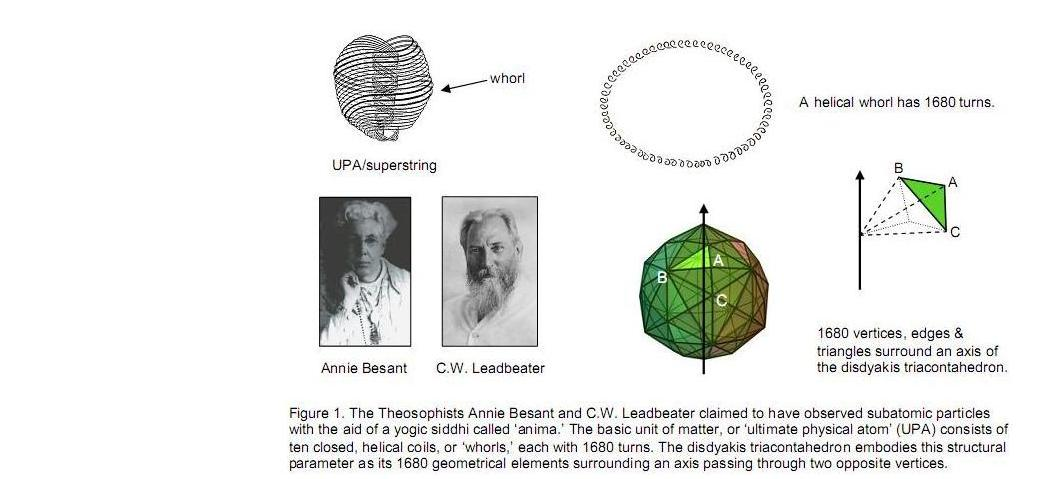outer sides, are divided into their sectors, 1680 vertices, lines & triangles surround an axis joining any pair of diametrically opposite vertices: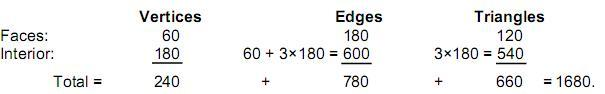The 1680 geometrical elements consist of 780 edges and 900 vertices & triangles. This 78:90 division is the polyhedral manifestation of the gematria number values (Table 1) of the Hebrew words Cholem and Yesodeth making up the name of the Kabbalistic Mundane Chakra of Malkuth, the Sephirah signifying the physical manifestation of the Tree of Life on a cosmic scale: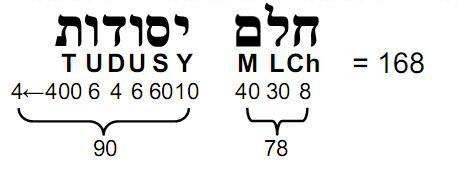This is a remarkable conjunction of three different contexts: the paranormal observation by Besant & Leadbeater of the basic units of matter, the gematria aspect of Kabbalah and the geometrical properties of the object that is the polyhedral counterpart of the universal Tree of Life blueprint (3). Even so, the diehard sceptic may feel tempted to dismiss it as coincidence — however implausible his suggestion may seem to more impartial minds. As a rebuttal, this article will demonstrate that the disdyakis triacontahedron also embodies the dimension 496 of the E8×E8 heterotic superstring gauge symmetry group. In other words, it will prove that the polyhedron embodies both the fundamental structural and

2

Table 1. Number values of the ten Sephiroth in the four Worlds.

 SEPHIRAH GODNAME ARCHANGEL ORDER OF ANGELS MUNDANE CHAKRA 1 Kether (Crown)                  620 EHYEH (I am)                  21 Metatron (Angel of the Presence)                  314 Chaioth ha Qadesh (Holy Living Creatures)                  833 Rashith ha Gilgalim First Swirlings. (Primum Mobile)                  636 2 Chokmah (Wisdom)                  73 YAHWEH, YAH (The Lord)                  26, 15 Raziel (Herald of the Deity)                  248 Auphanim (Wheels)                  187 Masloth (The Sphere of the Zodiac)                  140 3 Binah (Understanding)                  67 ELOHIM (God in multiplicity)                  50 Tzaphkiel (Contemplation of God)                  311 Aralim (Thrones)                  282 Shabathai Rest. (Saturn)                  317 Daath (Knowledge)                  474 4 Chesed (Mercy)                  72 EL (God)                  31 Tzadkiel (Benevolence of God)                  62 Chasmalim (Shining Ones)                  428 Tzadekh Righteousness. (Jupiter)                  194 5 Geburah (Severity)                  216 ELOHA (The Almighty)                  36 Samael (Severity of God)                  131 Seraphim (Fiery Serpents)                  630 Madim Vehement Strength. (Mars)                  95 6 Tiphareth (Beauty)                  1081 YAHWEH ELOHIM (God the Creator)                  76 Michael (Like unto God)                  101 Malachim (Kings)                  140 Shemesh The Solar Light. (Sun)                  640 7 Netzach (Victory)                  148 YAHWEH SABAOTH (Lord of Hosts)                  129 Haniel (Grace of God)                  97 Tarshishim or Elohim                  1260 Nogah Glittering Splendour. (Venus)                  64 8 Hod (Glory)                  15 ELOHIM SABAOTH (God of Hosts)                  153 Raphael (Divine Physician)                  311 Beni Elohim (Sons of God)                  112 Kokab The Stellar Light. (Mercury)                  48 9 Yesod (Foundation)                  80 SHADDAI EL CHAI (Almighty Living God)                  49, 363 Gabriel (Strong Man of God)                  246 Cherubim (The Strong)                  272 Levanah The Lunar Flame. (Moon)                  87 10 Malkuth (Kingdom)                  496 ADONAI MELEKH (The Lord and King)                  65, 155 Sandalphon (Manifest Messiah)                  280 Ashim (Souls of Fire)                  351 Cholem Yesodeth The Breaker of the Foundations. The Elements. (Earth)                  168

 The Sephiroth exist in the four Worlds of Atziluth, Beriah, Yetzirah and Assiyah. Corresponding to them are the Godnames, Archangels, Order of Angels and Mundane Chakras (their physical manifestation). This table gives their number values obtained by the ancient practice of gematria, wherein a number is assigned to each letter of the alphabet, thereby giving a number value to a word that is the sum of the numbers of its letters.

(All numbers in this table referred to in the article are written in boldface).

3

dynamical parameters of the E8×E8 heterotic superstring in a natural way, thereby rendering the possibility of coincidence as highly implausible.

2. The polyhedral basis of E8×E8 in the seven polygons
The 120 faces of the disdyakis triacontahedron are the sides of 30 pyramids. Their bases are the Golden Rhombic faces of a rhombic triacontahedron formed by the 20 C vertices of a dodecahedron and the 12 B vertices of an icosahedron, leaving 30 A vertices as their peaks. The vertices are arranged in either seven parallel sheets perpendicular to an axis passing through two opposite A vertices, 11 sheets perpendicular to an axis joining two opposite B vertices or 15 sheets perpendicular to an axis joining two opposite C vertices. Fig. 2 displays the projection of the vertices onto the central sheet. The topmost A vertex is at the centre of the graph in the XY plane.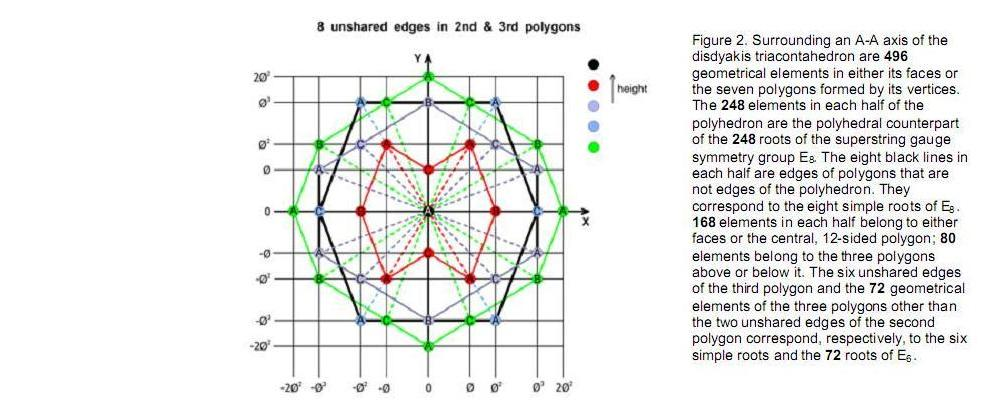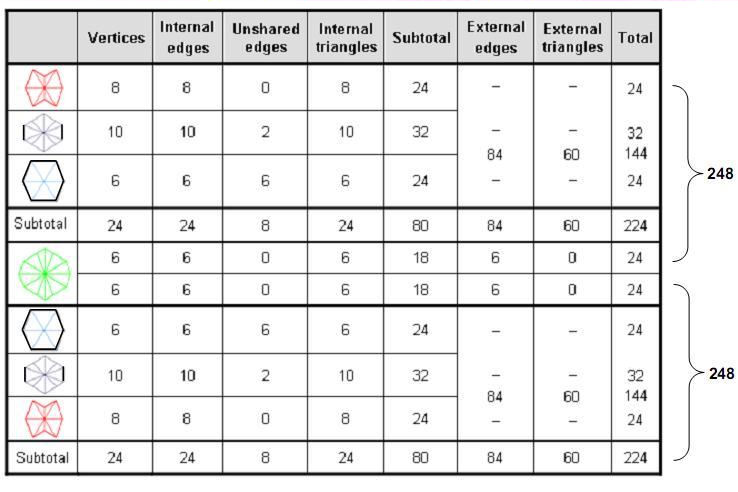4

Four types of polygons are formed by 36 vertices that are prescribed by ELOHA, Godname of Geburah with number value 36:

 8-sided polygons: 10-sided polygon: 6-sided polygon: 12-sided polygon: 4 A, 2 B & 2 C vertices 4 A, 2 B & 4 C vertices 4 A & 2 C vertices 4 A, 4 B & 4 C vertices.

The topmost three polygons have 24 vertices made up of 12 A vertices, four B vertices & eight C vertices; similarly for the lowest three polygons. The central polygon comprises four A vertices, four B vertices & four C vertices. The four types of polygons consist of 16 A vertices, eight B vertices & 12 C vertices. All seven polygons have 28 A vertices, 12 B vertices & 20 C vertices, with A vertices at the apex and nadir.

The numbers of geometrical elements in faces and interior polygons that surround an A-A axis of the disdyakis triacontahedron are set out below: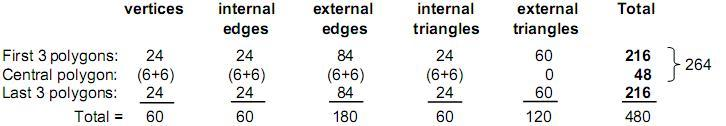The first three layers have 216 internal and external geometrical elements, where 216 is the number value of Geburah (see Table 1); similarly for the last three layers. Surrounding the centre of the central polygon are 48 elements (24 internal, 24 external), totalling 49 elements. This shows how EL ChAI, the Godname of Yesod with number value 49, prescribes the central polygon. 48 is the number value of Kokab, the Mundane Chakra of Hod. As explained in Article 40, it is a defining parameter of all holistic systems, as, indeed, is its 24:24 division displayed by the 24 geometrical elements in each half of the 12-sided polygon. Surrounding the A-A axis are 264 geometrical elements in the four layers of half the polyhedron, of which 36 are vertices, where 36 is the number value of ELOHA, the Godname of Geburah.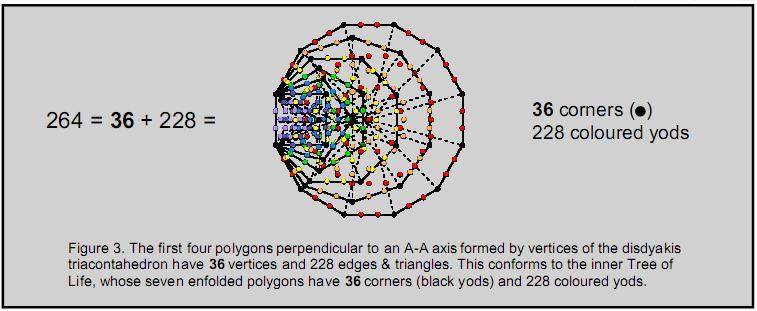Compare this with the fact that the seven enfolded polygons making a half of the inner Tree of Life have 36 vertices and 264 yods when their 47 sectors are tetractyses (Fig. 3). The number 264 defines half of the polyhedron, just as it does for its polygonal counterpart. The 12 vertices in the central polygon correspond to the 12 vertices of the first four enfolded polygons and the 24 vertices in the first three polygons correspond to the 24 vertices of the last three enfolded polygons.

The seven polygons defined by the vertices of the disdyakis triacontahedron have 480 geometrical elements made up of 240 elements and their 240 mirror images on the opposite side of the polyhedron. Each set of 240 elements consists of 168 elements in the faces (24 vertices, 60 triangles & 84 edges) above or below the plane of the central polygon and 72 elements that comprise six vertices and six external edges of the fourth polygon, 30 internal edges and 30 internal triangles. This 72:168 division reflects a particular division of roots of the superstring gauge symmetry group E8. The 72 elements correspond to the 72 roots of its subgroup E6 and the 168 elements correspond to the 168 roots of E8 that are not roots of E6. Inspection of Fig. 2 reveals that two edges of the 10-sided polygon in the second layer of vertices and all six edges of the six-sided polygon in the third layer are not edges of the disdyakis

5

triacontahedron, i.e., the two polygons have eight edges (denoted in Fig. 2 by black lines) that do not belong to the polyhedron. Their corresponding polygons below the XY plane similarly have eight unshared edges. Hence, the faces of the disdyakis triacontahedron and its seven polygons have in total 248 geometrical elements and their 248 mirror images, that is, 496 elements, 16 of which are polygonal edges that do not manifest as part of the form of the polyhedron. Its geometry therefore reflects the holistic pattern of the 496 roots of the E8×E8 heterotic superstring, the direct product of the two identical groups E8 with dimension 248 reflecting the inversion symmetry of the polyhedron, namely, the fact that every geometrical element has its mirror image. The eight unshared edges of polygons in each half correspond to the eight simple roots of each group. As the number value of Malkuth and the dimension of E8×E8, 496 is simply the number of geometrical elements in the faces of the polyhedron and in the seven polygons formed by its vertices that surround its A-A axis. This is the polyhedral basis of the heterotic superstring gauge symmetry group E8×E8. The property does not hold for any type of axis because, for example, in the case of the 15 polygons perpendicular to the C-C axis, none of their edges is an edge of the polyhedron. As we shall discover later, this configuration embodies not only the basic dynamical parameter of the E8×E8 heterotic superstring but also its basic structural parameter.

There are 30 vertices, 128 edges & 90 triangles in each half of the polyhedron, i.e., 128 edges and 120 vertices & triangles. One half geometrically encodes the forces between superstrings of ordinary matter, its mirror image half encodes the forces between superstrings of shadow matter. The 60 faces above the central plane are defined by 168 geometrical elements (84 edges, 84 vertices & triangles) that are analogous to the 168 circularly polarized oscillations made by each whorl of the UPA in a half-revolution, the 168 elements below the central plane corresponding to the 168 oscillations made in its other half-revolution — the mirror image of the first. The two sets of 60 faces, defined by two sets of 168 geometrical elements above and below the central plane, correspond to half-revolutions in the outer and inner halves of the UPA. The 168 ten-dimensional gauge fields corresponding to the 168 gauge charges of E8 that do not belong to E6 have 1680 space-time components. Is this the basis of the 1680 helical turns of each whorl? Not quite. In discussion in earlier articles of the inner Tree of Life basis of the 240 E8 gauge charges, wherein each whorl is represented by a Tree of Life, the first six enfolded polygons of whose inner form possess 24 corners denoting E8 gauge charges, it was suggested that each whorl carries 24, not 168, E8 gauge charges. In other words, the UPA has ten whorls simply because it possesses 240 E8 gauge charges distributed 24 to a whorl. That is what is indicated in the geometry of the inner form of ten overlapping Trees of Life that represent the ten whorls of the UPA, because 24 corners are associated with a set of the first six enfolded polygons, and this is repeated in each set enfolded in an overlapping Tree of Life, making 240 corners for the ten Trees of Life. Symbolizing independent degrees of freedom, these corners physically manifest as the 240 gauge charges and associated gauge fields of a superstring corresponding to the 240 roots of E8.

The seven polygons in the disdyakis triacontahedron have 60 internal edges surrounding the axis and 16 outer edges that are not polyhedral edges, i.e., 76 edges. YAHWEH ELOHIM, the Godname of Tiphareth with number value 76, prescribes the seven sheets of vertices. Adding the eight unshared edges to the 36 internal edges and 96 external edges in the first four polygons creates 140 edges. 140 is the number value of Masloth, the Mundane Chakra of Chokmah (also the number value of Malachim, the Order of Angels assigned to Tiphareth). 248 is the number value of Raziel, the Archangel of Chokmah.

There are nine vertices on the axis, namely, the centres of the seven polygons and the A vertices at the top and bottom. Hence, the total number of geometrical elements is (496+9=505). 504 elements surround the centre of the polyhedron. As we saw in Article 35 (4), this is the number of geometrical elements in the three-dimensional Sri Yantra when each of its 42 triangles is divided into its sectors! It is striking evidence that the Sri Yantra and the disdyakis triacontahedron are equivalent representations of divine archetypes. They are not identical in terms of their geometrical composition because the 504 elements in the polyhedron consist of 68 vertices, 256 edges & 180 triangles, whereas the 504 elements in the 42 primary triangles of the Sri Yantra comprise 126 vertices, 252 edges & 126 triangles (5). What counts as equivalence in this context is not mathematical isomorphism between corresponding geometrical elements but their total numbers, which are the same because, although dissimilar in their form, both sacred geometries embody numerically the same bits of information, spatially represented by points, lines and triangles. These three types of geometrical elements represent independent degrees of freedom. Any triangle exhibits seven of them: three vertices, three edges and the space inside it. The 120 triangular faces (Fig. 1) of the disdyakis triacontahedron are identical, each consisting of A, B & C vertices, edges AB, BC & AC and the triangle ABC. They play the role of the seven Sephiroth of Construction in the Tree of Life, which are similarly divided into two triads: Chesed-Geburah-Tiphareth & Netzach-Hod-Yesod and the single Sephirah, Malkuth. The three-fold nature of both vertices and edges reflects the active, passive & homeostatic pillars of the Tree of Life.

6

The Cosmic Tree of Life (CTOL) was discussed in Article 5 (6) as the Tree of Life of the spiritual cosmos. It consists of 91 overlapping Trees of Life with 550 Sephirothic levels (SLs). There are 504 SLs in CTOL down to the top of the 7-tree (the lowest seven Trees of Life) mapping the physical plane, the Malkuth level of CTOL. The centre of the polyhedron corresponds to the universe of space and time — the centre of the spiritual cosmos — mapped by the 7-tree. The 504 geometrical elements surrounding it are the counterpart of the 504 SLs that map superphysical levels of reality. There are 496 SLs down to the 55th SL from the bottom, which is Chesed of the ninth Tree of Life. The eight vertices on the A-A axis surrounding the centre of the polyhedron are the counterpart of the next eight SLs down to the top of the 7-tree. The 248 geometrical elements in one half of the polyhedron (they, of course, include half the central, 12-sided polygon) correspond to the 248 SLs in CTOL down to Binah of the 50th Tree of Life, which is prescribed by ELOHIM, Godname of Binah with number value 50 (see Table 1). The 248 mirror images of these elements, reflected through the centre of the polyhedron, correspond to the next 248 SLs down to Chesed of the ninth Tree of Life, whose Malkuth is the top of the 7-tree and the 25th tree level, marking the first of the 25 dimensions of the physical universe.

Adding the 16 (=42) unshared edges to the 240 edges listed above, the disdyakis triacontahedron has 496 geometrical elements surrounding its A-A axis that comprise 256 edges and 240 vertices & triangles, where 256 = 44, 240 = 1×2×3×4(1+2+3+4), and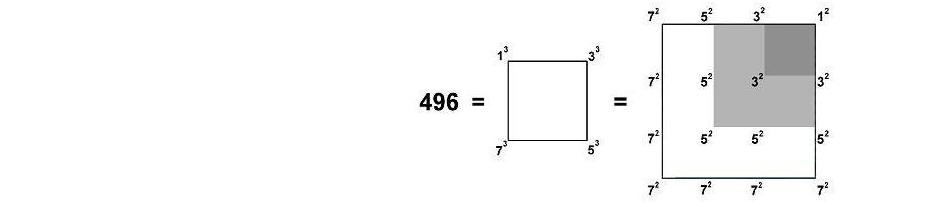This exemplifies how the Tetrad expresses the properties of holistic systems. As 496 = 16×31 = 16(1+15+15), we see that assigning the square of 4 to the 31 yods in a pentagon whose five sectors are tetractyses generates the 496 geometrical elements in the disdyakis triacontahedron, the central number, 42 = 16, denoting the number of unshared edges, the 15 internal squares of 4 adding to 240, which is the number of other edges, and the 15 squares of 4 on its boundary adding also to 240, the number of vertices & triangles. The Tetrad reveals a beautiful harmony between number and geometry that is always present in holistic systems like the disdyakis triacontahedron. The geometrical composition of the polyhedron is prescribed by EL, whose number value is 31 and by YAH, whose number value is 15. EL also prescribes the polyhedron because it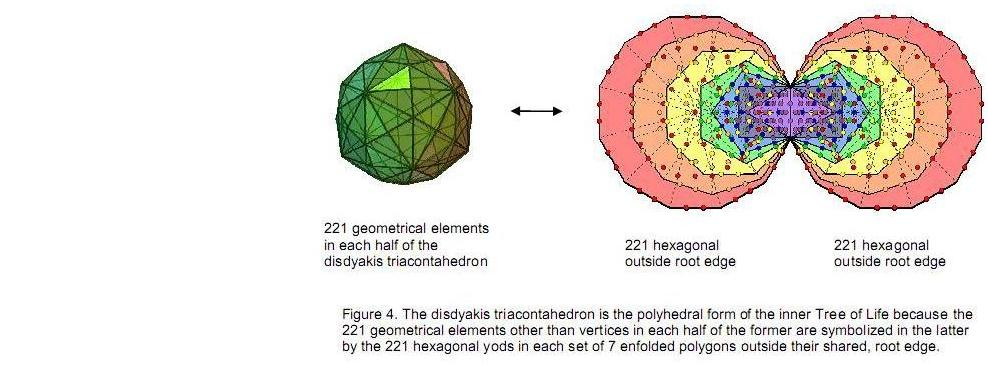has 31 vertices and their 31 mirror images.

Of the 504 elements surrounding its centre, 62 are polyhedral vertices, leaving 442 other elements. The

7

221 such elements in each half comprise 90 triangles, three centres of polygons & 128 edges, i.e., 131 vertices & edges. 131 is the number value of Samael, Archangel of Geburah. The (7+7) enfolded polygons of the inner TOL have 444 hexagonal yods (Fig. 4). This means that each set of seven enfolded polygons has 221 hexagonal yods outside the root edge, which has two hexagonal yods. They symbolize the extra 221 geometrical elements needed to construct the faces of the disdyakis triacontahedron and the seven polygons, starting from the vertices. The (7+7) polygons enfolded in each overlapping Tree of Life have 70 corners, of which 68 are outside the root edge. They comprise six corners that coincide with SLs of the outer Tree of Life (three on each side pillar), when the latter is projected onto the plane of the polygons, and 62 that belong solely to its inner form. The 62 unshared corners correspond to the 62 vertices of the disdyakis triacontahedron as the polyhedral version of the inner Tree of Life.

3. The embedding of E8×E8' in 10 Trees of Life
According to the table in Fig. 2, the 248 geometrical elements surrounding an A-A axis in each half of the polyhedron comprise 168 elements above the central polygon in its 60 faces and 80 other elements. The former comprise 24 vertices (12A, 4B & 8C), 84 edges (28 of each kind) and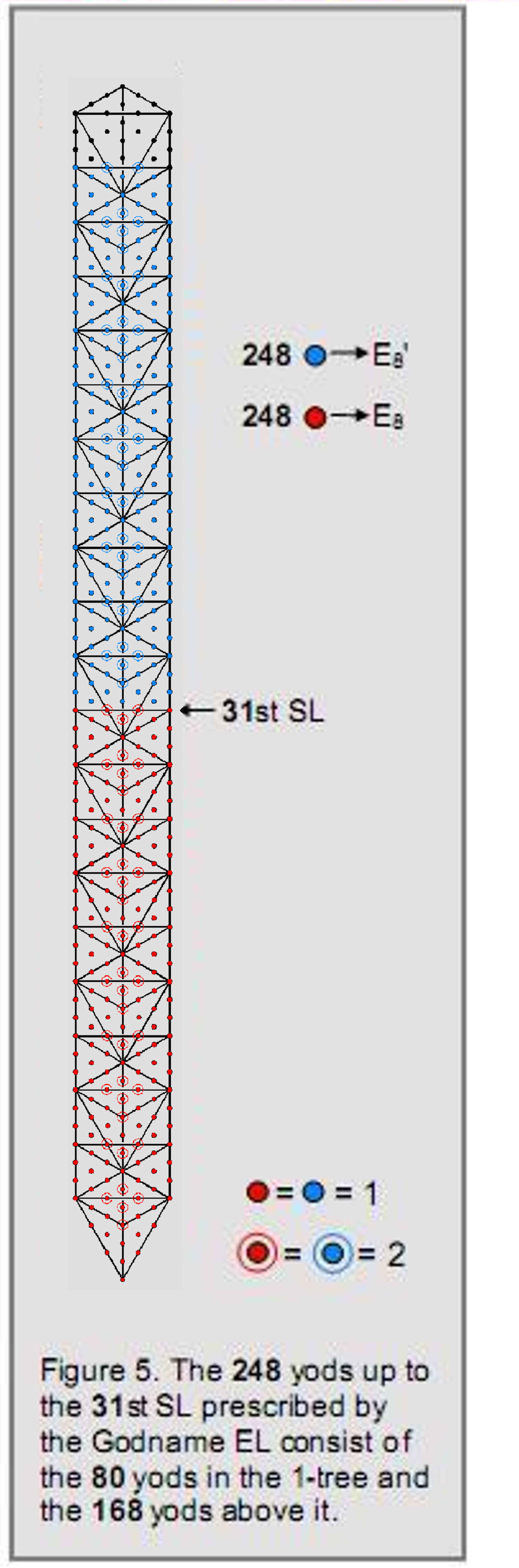60 triangles, i.e., 84 edges and 84 vertices or triangles. This 84:84 division in the superstring structural parameter 168 is characteristic of holistic systems in which it appears. The 80 elements are made up of the eight edges of polygons that are not polyhedral edges and 72 elements that comprise the six vertices in half the central polygon, 36 edges and 30 internal triangles. The latter correspond to the 72 roots of E6, one of the five exceptional groups. As stated earlier, the eight unshared edges correspond to the eight simple roots of E8. The six non-polyhedral edges of the six-sided polygon correspond to the six simple roots of E6 and the two non-polyhedral edges of the 10-sided polygon correspond to the two extra simple roots of E8.

The disdyakis triacontahedron exhibits the same division:

248 = 80 + 168,.

as is found for the 80 yods in the 1-tree and the 168 yods above it as far as Chesed of the fifth TOL, the 31st SL prescribed by EL, the Godname of Chesed (Fig. 5). The 80 elements comprise 30 internal triangles and 50 vertices & edges, where 50 is the number value of ELOHIM, Godname of Binah. This 30:50 division appears also in the 1-tree because the yod population of the n-tree is given by:

Y(n) = 50n + 30,

so that the (Y(1)=80) yods of the 1-tree comprise the 30 yods in its Lower Face and the 50 yods above it. Half of the polyhedron with 248 geometrical elements surrounding its axis is therefore the counterpart of the 248 yods in the 5-tree of CTOL up to its highest Chesed (the first Sephirah of Construction). Its mirror image half with 248 elements is the counterpart of the next 248 yods up to (but not including) Chesed of the tenth Tree of Life. As this is the 61st SL (again prescribed by EL because 61 is the 31st odd integer), the 496 yods contain 60 SLs as corners of triangles, just as the 496 geometrical elements include the 60 vertices of the polyhedron! The latter therefore are the counterpart of the SLs. The number of triangles in the n-tree is

T(n) = 16n + 9.

The 9-tree has (T(9)=153) triangles, where 153 is the number value of ELOHIM SABAOTH, Godname of Hod. There are three more triangles above the top of the 9-tree up to the 61st SL, so that the 496 yods belong to 156 tetractyses. As 156 is the 155th integer after 1, this shows how ADONAI MELEKH, the Godname of Malkuth with number value 155, prescribes the dimension 496 of E8×E8. ELOHA, the Godname of Geburah with number value 36, prescribes the dimension 248 of E8 because there are 36 hexagonal yods on the two side pillars up to the 31st SL. YAHWEH ELOHIM, Godname of Tiphareth with number value 76, prescribe this number because there are 76 tetractyses up to the 31st SL prescribed by EL.

8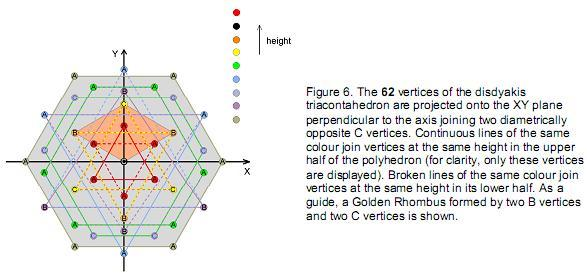4. Polyhedral embodiment of the superstring structural parameter 1680
We saw earlier that the disdyakis triacontahedron embodies the superstring structural parameter 1680 as the number of geometrical elements surrounding any axis when its internal triangles are divided into their sectors. As confirmation that this is not merely a coincidence, we found that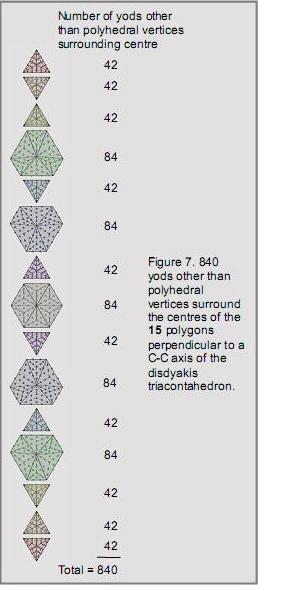the number is made of 780  edges and 900 vertices & triangles, reproducing (apart from a factor of 10) the gematria number values 78 and 90 of, respectively, Cholem and Yesodeth, the Kabbalistic name of the Mundane Chakra of Malkuth. It will now be shown how 1680 is the yod population of both the faces of the polyhedron and the 15 parallel polygons formed by its 60 vertices surrounding a C-C axis.

Fig. 6 displays the projection of the vertices of the polygons onto the XY plane occupied by the central polygon. They are triangles or 6-sided polygons, the central (eighth) polygon being a hexagon. Unlike in the case of the seven polygons perpendicular to an A-A axis discussed earlier, the vertices of a given polygon are of the same type. It means that none of their edges is an edge of the polyhedron. The significance of this is that, when the sectors of the polygons are turned into either tetractyses or divided into three tetractyses, none of the yods on the edges of polygons other than their vertices is shared with the polyhedron when its faces are similarly transformed. The only yods shared when its 180 internal triangles are turned into tetractyses are all vertices and the hexagonal yods on the internal edges of its sectors.

There are eight polygons in half the polyhedron with the following sets of vertices, commencing from the top: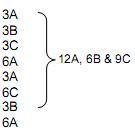The order in the polygons below the XY plane is reversed. The uppermost C vertex (black circle) at the origin of the XY coordinate system is below the level of the triangle formed by the three A vertices (red circles). The lowest C vertex is above their inverted counterpart.

9

Now suppose that the 60 sectors of the 15 polygons are divided into three tetractyses. The number of yods in an n-sided polygon with its sectors so divided is given by:

N(n) = 15n + 1.

(Notice that the number 15 of YAH, Godname of Chokmah, is the number of yods per sector). Triangles have (N(3) = 46) yods. 42 yods other than corners of the triangle surround its centre. A 6-sided polygon has (N(6)=91) yods. 84 yods other than corners surround its centre. There are 840 yods surrounding the centres of the 15 polygons other than their 60 vertices (Fig. 7). The five 6-sided polygons have 30 corners and (5×84=420) yods other than corners surrounding their centres, as do the ten triangles with 30 corners. This equal division of 420 yods is prescribed by the Godname YAH (YH) because the letter value 5 of H (heh) specifies the number of 6-sided polygons and the letter value 10 of Y (yod) specifies the number of triangles. This is yet more evidence of the archetypal nature of the disdyakis triacontahedron and its prescription by the Divine Names.

Suppose that the 120 faces of the disdyakis triacontahedron are turned into tetractyses. There is one hexagonal yod at the centre of each face and two hexagonal yods on each of its 180 edges. The number of yods in its faces surrounding the C-C axis = 60 +120 + 180×2 = 540. Every edge is also the side of an internal triangle with the centre of the polyhedron as one shared vertex. Next, suppose that the 180 internal triangles are turned into tetractyses. One hexagonal yod is at the centre of each tetractys and two hexagonal yods lie on each of the 62 internal edges ending on the vertices of the polyhedron. The number of yods in internal triangles that surround the C-C-axis = 180 + 60×2 = 300. Therefore, (540+300=840) yods surround this axis (this is true for any type of axis). The disdyakis triacontahedron is the Catalan solid with the most faces. Table 2 shows that, when all the Catalan solids with triangular faces are constructed from tetractyses, only the disdyakis triacontahedron has 840 yods surrounding an axis joining two diametrically opposite vertices:

Table 2. Various yod populations of the Catalan solids that have triangular faces.

 Catalan solid C E F C' Hs Hi H Ns Ni N triakis tetrahedron 8 18 12 6 48 30 78 54 30 84 rhombic dodecahedron - - - - - - - - - - triakis octahedron 14 36 24 12 96 60 156 108 60 168 tetrakis hexahedron 14 36 24 12 96 60 156 108 60 168 deltoidal icositetrahedron - - - - - - - - - - pentagonal icositetrahedron - - - - - - - - - - rhombic triacontahedron - - - - - - - - - - disdyakis dodecahedron 26 72 48 24 192 120 312 216 120 336 triakis icosahedron 32 90 60 30 240 150 390 270 150 420 pentakis dodecahedron 32 90 60 30 240 150 390 270 150 420 deltoidal hexacontahedron - - - - - - - - - - pentagonal hexacontahedron - - - - - - - - - - disdyakis triacontahedron 62 180 120 60 480 300 780 540 300 840

(C = number of vertices, E = number of edges, F = number of faces, C' = number of vertices surrounding the axis, Hs = number of hexagonal yods in faces, Hi = number of internal hexagonal yods surrounding the axis, H (=Hs+Hi ) = number of hexagonal yods surrounding the axis, Ns (=C'+Hs) = number of yods in faces surrounding the axis, Ni (= 2C'+E) = number of internal yods surrounding the axis & N (=Ni+Ns) = number of yods surrounding the axis.)

This is highly significant, because it indicates the unique status of this polyhedron in embodying the superstring structural parameters 840 and 1680, as would be expected if it were the polyhedral Tree of Life. The 15 polygons also have 840 yods other than vertices surrounding their centres. These, however, cannot be added to the 840 yods in the polyhedron that surround its axis because the latter include some of those in the central polygon, so that simply adding them together would involve some double counting. Two hexagonal yods on each internal edge of the six sectors of the hexagon are shared with the internal triangles of the polyhedron, although, as the outer edges of the hexagon connecting A vertices are not edges of the polyhedron, none of its sectors coincide with internal triangles of the latter — in fact, none of the sectors of polygons do, so that no other yods coincide. There are 12 hexagonal yods in the central polygon that are shared, as well as the 60 vertices and the centre of the polyhedron, i.e., 73 shared yods, where 73 is the number value of Chokmah. Of the 840 yods other than polyhedral vertices surrounding centres of the 15 polygons, (840–12=828) yods are unshared. Of the 840 yods belonging to the

10

polyhedron that surround the C-C axis, (12+60=72) yods are shared with polygons, leaving (840–72=768) unshared yods. Therefore, there are (828+768=1596) unshared yods. Four hexagonal yods belonging to the internal tetractyses of the polyhedron lie on the C-C axis, as do the 14 centres of the polygons and the two C vertices. These, too, are unshared. (1596+4+14+2=1616) yods are unshared and 73 yods are shared, totalling 1689 yods. 1680 yods surround the centre of the polyhedron other than the two C vertices at its poles and the six A vertices at its equator at the ends of shared edges. 840 yods belong to half the polyhedron; their 840 mirror images belong to its other half. They correspond to the 840 turns in the inner and outer halves of each helical whorl of the UPA. The Godname EHYEH with number value 21 prescribes the 21 yods on the axis. In fact, its letter values are the numbers of different yods:

AHIH = 21

 A = 1: H = 5: I = 10: H = 5: centre of polyhedron centres of five triangles above the central polygon two opposite A vertices + centres of four 6-sided polygons + four hexagonal yods centres of five triangles below the central polygon

This is true also for the letter values of the Godname YAH:

YH = 15

 Y = 10: centres of ten triangles above & below the central polygon H = 5: centres of five hexagons

This shows how both EHYEH and YAH prescribe the polygons.

As no C-C axis is unique, five hexagons with different A vertices at their corners can be chosen from the 30 A vertices in the disdyakis triacontahedron. This means that five axes can be drawn between opposite C vertices so that their associated central hexagons have no A vertices in common. Each similar set of 15 polygons has its own distinct set of 840 yods because none of them is a vertex of the polyhedron. In the case of an A-A axis, there are four A vertices in the central polygon, so that the six A vertices they form with the apex and nadir A vertices can be chosen in five ways from the 30 A vertices available Therefore, there are ten sets of polygons with 8400 yods. For each of the ten axes, there are 840 yods in the polyhedron, according to Table 2. Hence, there are ten copies of this primary set, that is, 8400 yods. The ten sets of polygons and their corresponding orientations of the polyhedron generate 16800 yods, which is the number of turns in the ten whorls of the UPA. The 8400 yods in the triangles of the ten copies of the polyhedron would correspond to the 8400 turns in the outer half of the UPA and the 8400 yods in the ten sets of polygons would correspond to the 8400 turns in its inner half. However, this has not taken into account the fact that some of the outer edges of the set of polygons perpendicular to an A-A axis are shared with the polyhedron, so that multiplying by 10 causes some overcounting. However, if it were meaningful for some reason to count copies separately, then the correspondence between the yod populations of the 110 polygons in the ten sets and the number of turns in all ten whorls of the UPA would be established. It is intriguing that the five choices of A axis and the five choices of C axis might be the counterpart in the Tree of Life of the first five Sephiroth and the last five Sephiroth, with the distinction between inner and outer halves of each whorl being due, simply, to mirror symmetry in the spatial distribution of yods. This issue will not be explored further here. The primary result has been established, namely, that 1680 yods surround the centre of the disdyakis triacontahedron apart from the two opposite C vertices and the six A vertices on its equator that lie on shared edges.

Table 3. Numbers of yods in the eight polygons in half the polyhedron (sectors as three tetractyses).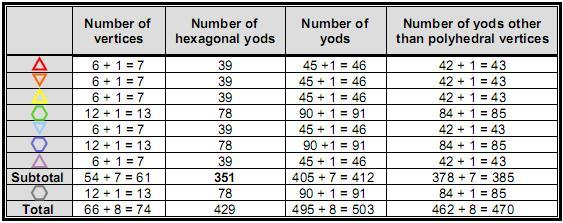11

The 840 yods surrounding the centres of the 15 polygons that are not polyhedral vertices consist of 60 vertices of 180 tetractyses and 780 hexagonal yods. Similarly, the 840 yods surrounding the axis of the polyhedron comprise 60 polyhedral vertices and 780 hexagonal yods. The 15 polygons have 900 yods surrounding the axis, when polyhedral vertices are included, whilst the polyhedron has 780 hexagonal yods. These two numbers cannot be added because the internal triangles of the latter share six edges with 12 hexagonal yods on them with the edges of the sectors of the hexagon. That apart, we see the same 90:78 division as found for the geometrical composition of the polyhedron.

5. Polyhedral embodiment of CTOL
Table 3 shows the numbers of yods in each polygon (‘1’ denotes the centre). The vertices refer to corners of tetractyses, not just to vertices of the polyhedron. The seven polygons above the XY plane have 61 vertices. This is prescribed by the Godname EL with number value 31 because 61 is the 31st odd integer. The hexagon has 13 vertices, so that the 15 polygons have (13 + 2×61 = 135) vertices. Including the top and bottom C vertices, we see that 137 vertices define the disdyakis triacontahedron and its 15 polygons. This is remarkable, because 137 is known to physicists as the integer whose reciprocal defines the fine-structure constant: α = e2/ħ c ≈ 1/137. In fact, the number 137 is a parameter of all holistic systems. For example, the inner form of the Tree of Life contains 1370 yods (the yods in 137 tetractyses) when the 94 sectors of its 14 enfolded polygons are constructed from three tetractyses (6). As 17 vertices lie on the C-C axis, there are (137–17=120) vertices surrounding it, where

120 = 22 + 42 + 62 + 82 .

This illustrates how the Tetrad Principle determines properties of objects possessing sacred geometry (8). Its counterpart in he inner Tree of Life is the 120 yods on the edges of the seven enfolded polygons. YAHWEH with number value 26 prescribes the seven polygons because they have 351 hexagonal yods, where 351 is the sum of the first 26 integers. They have 385 yods other than polyhedral vertices, where

 385 = 12 22 32 42 52 62 72 82 92 102 .

This demonstrates how the Pythagorean Decad, the measure of wholeness and perfection, determines the number of yods in the seven polygons generated by turning their sectors into three tetractyses. There are 66 vertices surrounding the centres of the eight polygons in half the polyhedron. As 66 is the 65th integer after 1, this property is prescribed by ADONAI, Godname of Malkuth with number value 65. 33 of them are polyhedral vertices, where 33 = 1! + 2! + 3! + 4!. 495 yods surround their centres. Including the C vertex at the top of the polyhedron, there are 496 yods other than the eight centres of the polygons in half the polyhedron, that is, 504 yods in total. Amazingly, as we found in Section 2, this is the number of geometrical elements in the polyhedron and the seven polygons that surround its centre! Moreover, it divides into the same two numbers: 8 & 496, for we found that 496 geometrical elements and eight points (two A vertices & six centres) on the A-A axis surround its centre! The geometrical composition of the whole polyhedron is symbolized by the yods making up the eight polygons and the C vertex. More important still, the 504 yods in half the polyhedron denote the 504 SLs in CTOL down to the top of the 7-tree whose 25 tree levels signify the 25 dimensions of space. This is the polyhedral counterpart of the three-dimensional Sri Yantra, whose 42 triangles contain 504 yods.

According to Table 2, the central hexagon has 91 yods and the two sets of seven polygons have (2×412=824) yods. Including the two opposite C vertices, there are 917 yods, so that 916 yods surround the centre of the polyhedron. This number is defined by the Tetrad and the Godname ELOHIM because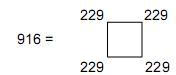where 229 is the 50th prime number. When n overlapping Trees of Life are constructed from tetractyses, the number of yods on the central Pillar of Equilibrium (9) is given by:

C(n) = 10n + 6.

The 91 overlapping Trees of Life of CTOL have (C(91)=916) yods on the central pillar. 49 overlapping Trees of Life have 496 such yods. The n-tree has

C'(n) = 10n + 10

yods (10), so that the 49-tree has (C'(49) = 500 = 50×10) yods, indicating how ELOHIM with number value

12

50 prescribes the seven planes of consciousness mapped by the 49-tree as well as CTOL itself. Inspection of Fig. 5 shows that there are three yods on the central pillar between Daath of any Tree of Life and the top of the next lower one. Therefore, there are 503 yods below Daath of the 50th Tree of Life. The 916 yods surrounding the centre of the polyhedron correspond to the 916 yods on the central pillar of CTOL and the 503 yods in the polygons of half the polyhedron correspond to the 503 yods below Daath of the 50th Tree of Life. Because Binah of the 50th Tree of Life is the 248th SL from the top of CTOL, Daath of the 50th Tree of Life is the first of the next 248 SLs down to the 496th one — Chesed of the ninth Tree of Life. In other words, the 412 yods above the central plane of the polyhedron define the split of the superstring number 496 into the two 248s of E8×E8', the domain with 412 yods above the central polygon corresponding to the shadow matter group E8' and the lower half of the polyhedron with 504 yods (including the lowest C vertex) corresponding to the ordinary matter group E8. As a counterpart of CTOL, the lowest eight polygons and the lowest C vertex can be thought of as representing the 504 yods up to (and including) Daath of the 50th Tree of Life prescribed by ELOHIM, Godname of Binah with number value 50. This SL marks the commencement of the second group of 248 SLs emanating from Binah of the 50th Tree of Life, the 248th SL from the top of CTOL. The next 412 yods in the seven upper polygons correspond to the next 412 yods on the central pillar up to the top of CTOL. This correspondence requires us to count the centre of the central polygon as included in the 916 yods because it is among the 503 yods in the eight lowest polygons, so that the 916 yods in question are not those surrounding this point but, rather, those below the topmost C vertex, with the lowest C vertex corresponding to the lowest point of CTOL. The eight centres of the eight lowest polygons correspond in CTOL to the four yods above the top of the 49-tree to Daath of the 50th Tree of Life and to the four yods added by turning 49 overlapping Trees of Life into the 49-tree. The 495 yods surrounding their centres correspond to the 495 yods on the central pillar up to, and including, Daath of the 49th Tree of Life.

914 yods in the 15 polygons surround the centre of the central hexagon. This is the number of hexagonal yods lining the 457 edges of the 282 tetractyses in the 14 enfolded polygons of the inner Tree of Life when their sectors are divided into three tetractyses (11). It is more remarkable evidence for the Tree of Life nature of the disdyakis triacontahedron. 282 is the number value of Aralim, the Order of Angels assigned to Binah (see Table 1). 457 is the number of yods in half the polyhedron. Each half of the inner Tree of Life has 229 edges (the root edge being shared). As pointed out earlier, 229 is the 50th prime number, showing how ELOHIM prescribes each half.

Geometrical counterpart of CTOL
Let us now examine the geometrical elements that are intrinsic to the 15 polygons, i.e., unshared with the polyhedron, when their sectors are divided into three triangles. They are shown below: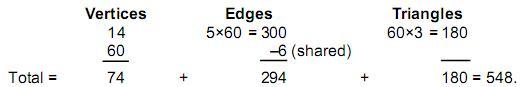548 unshared elements in the 15 polygons surround the centre of the polyhedron, which they share with it . Including the two C vertices, which the polyhedron does not share with the polygons, there are 550 unshared elements surrounding the centre, consisting of 76 vertices, 294 edges and 180 triangles, that is, 474 edges & triangles. 76 is the number value of YAHWEH ELOHIM, Godname of Tiphareth, and 474 is the number value of Daath (“knowledge”). The 550 geometrical elements are on the C-C axis or unshared with the polyhedron. This is the geometrical counterpart of the 550 SLs of CTOL. There are 474 SLs down to the top of the twelfth Tree of Life, whose Malkuth is the 65th SL prescribed by ADONAI because it is the top of the 10-tree, which embodies information about the physics of the E8×E8 heterotic superstring, being the Tree Life representation of its ten space-time dimensions. The 76 vertices in the 14 polygons or on the C-C axis are the counterpart of the 76 SLs below the top of the twelfth Tree of Life.

The 14 polygons above and below the central hexagon have 54 internal vertices, 14 centres, 270 edges & 162 triangles, totalling 500 (=50×10) geometrical elements. This shows how ELOHIM, Godname of Binah with number value 50, prescribes the 14 polygons. Each set of seven polygons has 250 elements. Including the two C vertices, there are 251 elements below the central hexagon, which has 48 unshared elements surrounding its centre, and 251 elements above it. In other words, there are (251+48=299) geometrical elements in the eight polygons making up half the polyhedron other than its centre and 251 elements above it. In terms of the correspondence with the 550 SLs of CTOL, this is remarkable, for there are 299 SLs up to the top of the 49-tree mapping the seven planes of consciousness and 251 SLs in the 42 Trees of Life above it (12). What this means is that the topmost C vertex represents the top SL of CTOL, the lowest vertex represents the lowest SL and the 48 elements in the central polygon represent the next 48 SLs up to Chesed of the eighth Tree of Life, which is the 49th SL prescribed by EL ChAI,

13

Godname of Yesod, whose meaning, “foundation,” is appropriate, given that there are 25 tree levels up to this point in CTOL representing the 25 spatial dimensions of the universe. In correlating the elements in the 14 polygons with SLs, we have to start counting from the lowest C vertex, proceed to the central polygon, then count elements in the lower set of seven polygons and, finally, elements in the upper set. It is truly remarkable that exactly half the polyhedron should correspond to the seven planes of the cosmic physical plane mapped by the 49-tree and that the upper half should correspond to the six cosmic superphysical planes mapped by the next 42 Trees of Life making up CTOL. This is further, spectacular evidence that the polygons defined by the C-C axis constitute a map of CTOL — this time not in terms of their yods correlating with the yods on the central Pillar of Equilibrium of CTOL but in terms of their geometrical elements correlating with its SLs. Table 3 indicates that the eight lowest polygons in the polyhedron have 496 yods other than centres surrounding its centre when the lowest C vertex is added. This is consistent with 496 being the number value of Malkuth, whose cosmic manifestation is the cosmic physical plane with 299 SLs represented by these polygons in the lower half of the polyhedron.

Lambda Tetractys pattern
Let us next consider just the 15 polygons and the outer form of the polyhedron, ignoring the internal triangles of the latter. This means that the six internal edges of the hexagon need no longer be considered shared. In this case, the 15 polygons have 555 geometrical elements (75 non-polyhedral vertices, 300 edges & 180 triangles), whilst the polyhedron has 62 vertices, 180 edges and 120 triangles, i.e., 362 geometrical elements. Hence, the polyhedron and its polygons have (362+555=917) geometrical elements (137 vertices, 480 edges & 300 triangles). Remarkably, this is the same as the number of yods on the axis and in the 15 polygons when their sectors are each constructed from three tetractyses! 17 vertices lie on the C-C axis, so that 900 geometrical elements surround it. They comprise the 60 vertices of the polyhedron, the 480 edges of their sectors and the 360 internal vertices & triangles. This 60:480:360 pattern is the division displayed by the Lambda Tetractys discussed in previous articles (13):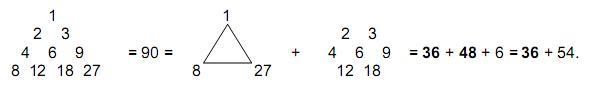It is convincing evidence that the polyhedron and its internal polygons constitute an archetypal object of sacred geometry. Fittingly, the 60 polyhedral vertices correspond to the number 6 at the centre of the tetractys, the yod symbolizing Malkuth, for these shape-determining vertices are the Malkuth aspect of the polyhedron. The same 36:54 pattern is exhibited in the disdyakis triacontahedron when its faces and internal triangles are divided into their sectors, for there are, then, (120×3=360) triangles in its faces and (180×3=540) internal triangles, totalling 900 triangles. Musically, the ratio of these numbers: 540/360 = 3/2 defines the interval of a perfect fifth. As Goethe said, “Geometry is frozen music.”

6. The polyhedral basis of E8×E8 in the 15 polygons
The 550 SLs of CTOL consist of the 496 SLs down to Chesed of the ninth Tree of Life and the next 54 SLs. Identifying the former as being the counterpart of the 496 gauge bosons of E8×E8' enables us to correlate with the root structure of this group the geometrical composition of the 15 polygons and the two C vertices, which consist of 550 unshared elements surrounding the centre of the disdyakis triacontahedron, as now explained. Below are displayed these geometrical elements: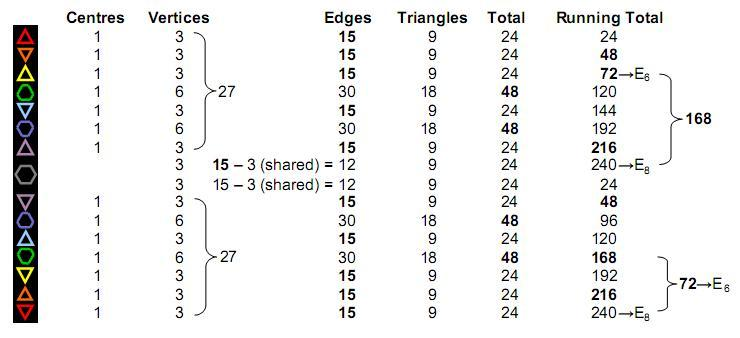14

We see that, apart from the 27 vertices in the seven upper or lower polygons, there are 237 edges & triangles and three vertices in the central hexagon, i.e., 240 unshared geometrical elements. They correspond to the 240 roots of E8'. The seven centres of the upper polygons and the top C vertex correspond to its eight simple roots. The seven lower polygons and the other half of the hexagon have 240 unshared geometrical elements corresponding to the 240 roots of the second group E8. The seven centres of the lower hexagons and the lowest C vertex correspond to the eight simple roots of E8. The reason for associating the upper half with the second group E8' rather than the first group E8 is that superstrings of shadow matter are far less detectable than those of ordinary matter, so that it is apt that their gauge symmetry group should be associated with the upper half of the polyhedron, which corresponds to the cosmic superphysical region of CTOL.

What about the 54 vertices inside the sectors of the polygons? Together with the lowest C vertex, they are the counterpart of the 55 SLs up to the 496th SL from the top of CTOL. Counting down from the top C vertex and ignoring internal vertices, the 248th geometrical element is the last of those in half the central hexagon. It corresponds to Binah of the 50th Tree of Life specified by its Godname, ELOHIM. The next 248 elements take us down to the lowest C vertex, which corresponds to the 55th SL, Chesed of the ninth Tree of Life. As the lowest 26 Trees of Life in CTOL have a correspondence to the lowest 26 tree levels signifying the 26 dimensions of space-time predicted by quantum mechanics for bosonic strings, this SL corresponds to the ninth spatial dimension. In other words, this parallel is indicating that the 496 gauge bosons that correspond to the 496 SLs in CTOL down to that point manifest in a space-time with nine spatial dimensions, i.e., ten-dimensional superstring space-time. Malkuth — the physical universe — requires nine spatial dimensions, according to its gematria number value, for both fermions and bosons to co-exist.

The lowest three triangles have 72 edges & triangles (45 edges, 27 triangles). This is the number of roots in its exceptional subgroup E6. The centres of the five triangles and a centre of one 6-sided polygon correspond to its six simple roots. The next four polygons have 168 vertices, edges & triangles that correspond to the 168 roots of E6 that are not roots of E6. They comprise 90 edges of these polygons and 78 vertices, edges & triangles. These are the gematria number values of Yesodeth and Cholem in the Hebrew name of the Mundane Chakra of Malkuth (p. 2). Such matching between the numbers of geometrical elements and the values of individual words strongly argues against coincidence, supposing that such an argument were still needed. The 45 edges in the three lowest triangles and the 81 triangles in the seven polygons correspond to the 126 roots of E7. Its seven simple roots correspond to the centres of the seven polygons. The lowest C vertex is the counterpart of the extra simple root of E8. Similar correspondences for the roots of E8' apply in the upper seven polygons.

Embodiment of the number of Tiphareth (“Beauty”)
The disdyakis triacontahedron has 180 edges, 120 triangles, 180 internal triangles and 60 internal edges surrounding its axis. Six internal edges are internal edges of the central hexagon, which are shared with that of the polyhedron, so that it has 534 unshared geometrical elements surrounding its axis. Other than the two edges making up the axis, the polyhedron possesses 534 unshared elements surrounding its centre. As we saw earlier, the 15 polygons have 548 unshared elements. The polygons and polyhedron have (534+548=1082) unshared elements other than those forming its axis. This is the 1081st integer after 1, which for the ancient Pythagoreans was not an integer but the Monad, the source of all number, so that they would have considered the number 1082 as really the 1081st integer. 1081 is the gematria number value of Tiphareth, the Sephirah at the centre of the Tree of Life (Table 1). The number value of the Hebrew word meaning “beauty” quantifies the geometrical degrees of freedom of the polyhedron and its 15 polygons. This mathematical beauty is that of the polyhedral representation of the divine archetype governing the form and structure of holistic systems such as CTOL and the whorl of the E8×E8' heterotic superstring.

References
1.The Catalan solids are the duals of the more well-known Archimedean solids (polyhedra with two or more types of regular polygons as faces), wherein the faces of the latter become vertices and their vertices become faces.
2. Numbers in Table 1 that appear in the text are written in boldface.
3. Phillips, Stephen M. Article 22: “The disdyakis triacontahedron as the three-dimensional counterpart of the inner Tree of Life,” (WEB, PDF).
4. Phillips, Stephen M. Article 35: “The Tree of Life nature of the Sri Yantra and some of its scientific meanings,” (WEB, PDF), Table 3, pp. 7, 8.

15

5. Ibid, p. 7.
6. Phillips, Stephen M. Article 5: “The superstring as microcosm of the spiritual macrocosm,” (WEB, PDF), p. 2 et seq.
7. Ref. 4, p. 10.
8. Phillips, Stephen M. Article 1: “The Pythagorean nature of superstring and bosonic string theories,” (WEB, PDF), p. 5.
9. Fig. 5 indicates that there are ten yods on the central pillar up to, and including, Tiphareth of the 1-tree. Then there are ten yods added in successive upper Faces. Therefore, the number of yods on the central pillar of the (n–1)-tree with (n–1) more Faces = 10(n–1) + 10 = 10n. For n overlapping Trees of Life, there are six yods on this pillar above the (n–1)-tree. The total number of yods on the central pillar of n overlapping Trees of Life = 10n +6.
10. Ten yods on the central pillar are added to the ten yods in the lowest Face by each Upper Face of the next higher Tree of Life. The number of yods on the central pillar of the n-tree = 10n + 10.
11. There are 1370 yods in the (7+7) enfolded polygons of the inner Tree of Life when their 94 sectors are divided into three tetractyses. These sectors have 80 vertices. Their division adds 94 vertices of tetractyses, so that the total number of vertices is (80+94=174). Hence, there are (1370–174=1196) hexagonal yods. A hexagonal yod is at the centre of each of the (94×3=282) tetractyses. Therefore, there are (1196–282=914) hexagonal yods lining the (914/2=457) edges of these tetractyses.
12 For more details about the significance of the number 251 vis-à-vis CTOL, see ref. 6.
13. Phillips, Stephen M. Article 30: “The equivalence of the triakis tetrahedron, disdyakis triacontahedron and Plato’s ‘Lambda Tetractys,’” (WEB, PDF), p. 6.

16

Home